## The definition of area under curve

Let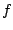be a continuous function on interval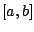. Divideinto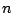subintervals of length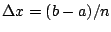. Choose (sample) points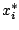in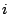th interval, for each. The (signed) area between the graph ofand the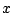axis is approximately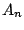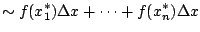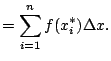(The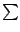is notation to make it easier to write down and think about the sum.)

Definition 2.1.1 (Signed Area)   The (signed) area between the graph ofand theaxis between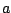and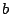is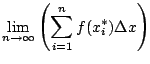(Note thatdepends on.)

It is a theorem that the area exists and doesn't depend on the choice of.

William Stein 2006-03-15